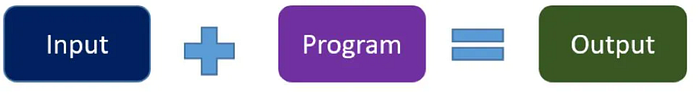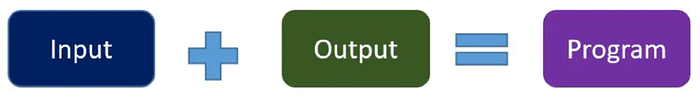23 Common Data Science Interview Questions for Beginners
Career · 9 Feb 2023, 10:07 · 12 mins read ·
6

In 2012, Harvard Business Review called data scientists the sexiest job of the 21st century. However, correctly answering data science interview questions to get a job as a data scientist is very tricky, and great preparation is the best way to ace the interview.

During the interview, the interviewer can ask questions from different data science topics such as statistics, programming, data analysis, data pre-processing and modeling. Your skills will be put to the test, and you need to prepare yourself if you want to pursue a career in data science. In this article, I have compiled a list of common data science interview questions with guides on how you can answer them, and a list of resources to learn more about the specific topic presented in each interview question.

### 1. What is Logistic Regression? State an example of when you have used Logistic Regression recently

Logistic regression is a popular algorithm used to solve classification problems. In this question, you need to explain what logistic regression is, how it works, and an example of a data science problem you solved by using logistic regression.

### 2. Why do we need Evaluation Metrics? What is a confusion matrix?

Machine learning models must be evaluated to check their performances. In this question, you need to explain how you can use the confusion matrix to evaluate the model performance. You can further mention other metrics to evaluate regression and classification models.

### 3. How is Data Science Different from Traditional Application Programming?

A good way to answer this question is by using examples of how the program is created in both cases. Traditional programming approach"📷

Data Science approach:📷

### 4. Explain the Difference Between Supervised and Unsupervised Learning.

Supervised and unsupervised learning are among the types of machine learning techniques. The best way to answer this question is by explaining their differences in terms of the kind of datasets you can use in each technique and examples of algorithms.

### 5. What is a Decision Tree?

A decision tree is another supervised learning algorithm that can be used to solve regression or classification problems. It is recommended to explain how the decision tree algorithm learns from the data and the advantages and disadvantages of using a decision tree algorithm.

### 6. What is Cross-Validation?

The purpose of the question is to determine if you know some techniques used to assess the effectiveness of the machine learning model. For example, when you want to avoid overfitting. When answering this question, it is recommended to explain some methods of cross-validation you have applied in any data science projects.

### 7. What is a Normal Distribution?

This term is commonly used when solving a data science problem. In this question, you can explain the meaning of normal distribution, its properties, and why it is important to check if your data is normally distributed.

### 8. What is a Random Forest Algorithm?

Random forest is one of the popular machine learning algorithms. When answering this question, it is recommended to explain how the algorithm learns from the data and when it is recommended to use the random forest algorithm over other machine learning algorithms.

### 9. Explain Univariate, Bivariate, and Multivariate Analyses

These three types of analyses are used to summarize variables in the dataset and help you get some insights. You can also share their differences and when you can apply them with examples.

### 10. How would you handle missing data?

Some datasets may have missing data or values and can cause a problem when training machine learning models. It is important to mention some techniques that can be used to handle missing data. You can also share your experience of how you handle missing data in your last data science project.

### 11. What is the benefit of dimensionality reduction?

Dimensionality reduction is the technique to reduce the number of features or variables in the dataset. There are different advantages or benefits of dimensionality reduction you can explain when answering this question. It is recommended to explain why and when you need to apply this technique.

### 12. How would you deal with outliers in a dataset?

An outlier is a data point that deviates significantly from the rest. In this question, you can explain how one can identify outliers and different techniques used to deal with outliers.

### 13. What is Ensemble Learning?

In machine learning, ensemble learning is a process of using multiple algorithms to obtain better predictive performance than could be obtained from any algorithms alone. When answering this question, you can also share your experience the last time you implemented ensemble methods in a data science project.

### 14. Explain how Machine Learning is different from Deep Learning?

The best way to explain the difference between machine learning and deep learning is the way they solve problems. You can go further by explaining some of the problems that can be solved by either machine learning or deep learning techniques.

### 15. What are the differences between Overfitting and Underfitting?

The best way to explain the difference is not just with definition but through examples. You can also share your personal experience when faced with overfitting or underfitting problems in a data science project.

### 16.What is Regularisation? Why is it useful?

When answering this question, you can also go further by explaining the two common regularization techniques L1 norm and L2 norm.

### 17. What is Selection Bias?

It is not enough to define Selection Bias. If possible you can explain different types of bias, their effects, and how to avoid them.

### 18. Can you explain the difference between a validation set and a test set?

In this question, after explaining their differences, you can explain the advantage of having a validation set and a test set in a data science project.

### 19. What is the difference between Regression and Classification ML techniques?

We all know that regression and classification are supervised learning and the only difference is their output. When you answer this question, you can mention a few algorithms that can be used to solve regression problems or classification problems. Also, try to share how their models are evaluated.

### 20. What are Artificial Neural Networks?

In this question, don’t just define Artificial Neural Networks, but also explain their advantages and where you can use them.

### 21.What tools and devices do you plan to use in your role as a Data Scientist?

This question is straightforward but it is recommended to mention tools you have used before or you are planning to use in the future project. You can also share your experience of how the tools help you implement the data science project successfully.

Keep in mind, you will use different tools for different projects. For example, some tools can be used for an NLP project and others for a time-series project.

### 22.What is Natural Language Processing (NLP)? State some real-life examples of NLP.

You have to define Natural language processing in a simple way and how it can be used to solve business problems. Then share some real-life examples. If possible you can also share some of the NLP projects you have done or collaborate with others.

### 23. What is Normalisation? Do you know the difference between Normalisation and Standardisation?

Normalisation and standardisation are techniques used to pre-process the data before applying machine learning algorithms. The purpose of the question is to explain the differences between these two techniques and at what condition of the dataset, you should apply one over another.

### Final Thoughts on Data Science Interview Questions

Reviewing these common data science interview questions will actually boost your confidence during the interview. Don’t expect the interviewer to ask you all questions mentioned in this article. However, most of the interview questions will come from the same topics.

For example, instead of asking “Explain the difference between supervised and unsupervised learning”. The interviewer can ask you to “Explain some supervised learning algorithms and how they learn from the data”.

If you are interested in learning and reading more data science interview questions, I recommend you take your time to read the following resources I have compiled for you.

It is recommended to practice your coding skills because some questions during the interview require you to code the solution. I hope these data science interview questions will help you in preparing for your interview and I wish you the best of luck in your data science career.

If you learned something new or enjoyed reading this article, please share it so that others can see it. Until then, see you in the next post!